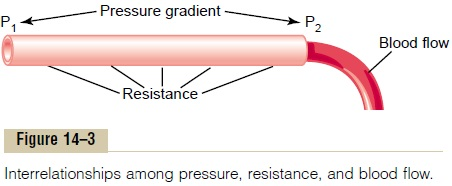Home | | Medical Physiology | Interrelationships Among Pressure, Flow, and Resistance

# Interrelationships Among Pressure, Flow, and Resistance

Blood flow through a blood vessel is determined by two factors: (1) pressure difference of the blood be-tween the two ends of the vessel, also sometimes called “pressure gradient” along the vessel, which is the force that pushes the blood through the vessel, and (2) the impediment to blood flow through the vessel, which is called vascular resistance.

Interrelationships Among Pressure, Flow, and Resistance

Blood flow through a blood vessel is determined by two factors: (1) pressure difference of the blood be-tween the two ends of the vessel, also sometimes called “pressure gradient” along the vessel, which is the force that pushes the blood through the vessel, and (2) the impediment to blood flow through the vessel, which is called vascular resistance. Figure 14–3 demonstrates these relationships, showing a blood vessel segment located anywhere in the circulatory system.P1 represents the pressure at the origin of the vessel; at the other end, the pressure is P2. Resistance occurs as a result of friction between the flowing blood and the intravascular endothelium all along the inside of the vessel. The flow through the vessel can be cal-culated by the following formula, which is called

Ohm’s law:in which F is blood flow, ΔP is the pressure difference (P1 - P2) between the two ends of the vessel, and R is the resistance. This formula states, in effect, that the blood flow is directly proportional to the pressure dif-ference but inversely proportional to the resistance.

Note that it is the difference in pressure between the two ends of the vessel, not the absolute pressure in the vessel, that determines rate of flow. For example, if the pressure at both ends of a vessel is 100 mm Hg and yet no difference exists between the two ends, there will be no flow despite the presence of 100 mm Hg pressure.

Ohm’s law, illustrated in Equation 1, expresses the most important of all the relations that the reader needs to understand to comprehend the hemodynam-ics of the circulation. Because of the extreme impor-tance of this formula, the reader should also become familiar with its other algebraic forms:

DΔ = F x RStudy Material, Lecturing Notes, Assignment, Reference, Wiki description explanation, brief detail
Medical Physiology: Overview of the Circulation; Medical Physics of Pressure, Flow, and Resistance : Interrelationships Among Pressure, Flow, and Resistance |

Related Topics